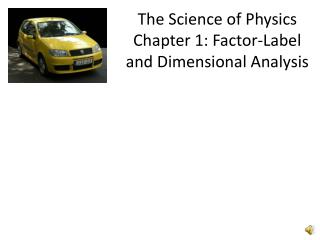Download PresentationThe Science of Physics Chapter 1: Factor-Label and Dimensional AnalysisThe Science of Physics Chapter 1: Factor-Label and Dimensional Analysis - PowerPoint PPT Presentation

Download PresentationThe Science of Physics Chapter 1: Factor-Label and Dimensional Analysis
An Image/Link below is provided (as is) to download presentation

Download Policy: Content on the Website is provided to you AS IS for your information and personal use and may not be sold / licensed / shared on other websites without getting consent from its author. While downloading, if for some reason you are not able to download a presentation, the publisher may have deleted the file from their server.

- - - - - - - - - - - - - - - - - - - - - - - - - - - E N D - - - - - - - - - - - - - - - - - - - - - - - - - - -
Presentation Transcript

1. The Science of PhysicsChapter 1: Factor-Label and Dimensional Analysis

2. Factor Label Method • Why unit conversions? • Want all numbers to have like units before performing calculations • May want to represent number differently

3. Factor Label Method • Need conversion factor • Conversion factor is equal to 1 so doesn’t change amount of what you have • Example: convert 32 inches to feet • Conversion factor: 1 foot = 12 inches or 1 ft/12 in • Convert– 32 inches x (1 ft/12 inches) = 2.7 ft • Not 3 ft and not 2.6667 ft !

4. Factor Label Practice Handout

5. Dimensional Analysis • Dimensional Analysis is a tool to see if you’ve set up your equation correctly. • For instance, say you want to know how long it will take you to travel to Iowa City which is about 75 miles away and you know you will be travelling at an average speed of 50 miles per hour.

6. How to solve problem using dimensional analysis… 1. Identify equation to use: D= s x t D= distance travelled, miles S = speed, miles per hour t = time travelled, hours 2. Rearrange equation such that unknown is isolated on one side: t = D/s 3. Substitute values with units and solve: t = 75 miles/ (50 miles/hour) = 75 miles x (hour/50 miles) = 1.5 hours

7. How to solve problem using dimensional analysis…oops! 1. Identify equation to use: D= s x t D= distance travelled, miles S = speed, miles per hour t = time travelled, hours 2. Rearrange equation such that unknown is isolated on one side: t = D x s 3. Substitute values with units and solve: t = 75 miles x (50 miles/hour) = 3750 miles2/hour